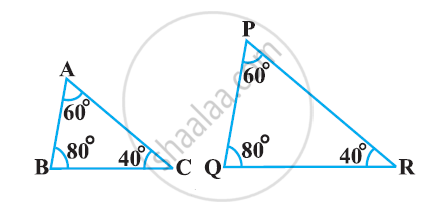Share

# State which pair of triangles in the following figure are similar? Write the similarity criterion used by you for answering the question and also write the pairs of similar triangles in the symbolic form - Mathematics

Course

#### Question

State which pair of triangles in the following figure are similar? Write the similarity criterion used by you for answering the question and also write the pairs of similar triangles in the symbolic form:#### Solution

∠A = ∠P = 60°

∠B = ∠Q = 80°

∠C = ∠R = 40°

Therefore, ΔABC ∼ ΔPQR [By AAA similarity criterion]

Is there an error in this question or solution?